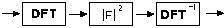## Doing an autocorrelationThe autocorrelation of the input image, `input`, can be constructed from the command

```» out = auto(input);
```

where `auto` is the following Matlab file

function f = auto(a)
%
% AUTO
% f = auto(a)
% returns as f the auto-correlation of image a.

f = fft2(a);
f = f.*conj(f);
f = ifft2(f);
f = fftshift(f);
f = real(f);
f = f/max(max(f));

Maintained by John Loomis, last updated 8 March 2000